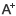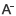# 苏教版小学六年级上册数学寒假作业答案【9-19页】日期：2020-12-10     来源：www.dazhixue.com    作者：智学网    浏览：595    评论：0

第 9 页
1） 1/20 1 1/4 7/3 2 3/7 12 1/6 9 5
2） （1） 8/9 9 1/9
（2） 3/ 2 ÷3/8=4 3/2 ÷4=3/8
（3） 4/3 1/12
（4） > > < < = <
3） （1） × （2） × （3） × （4） √
第 10 页
4）（1） A （2） B （3） C （4） C
5）（1） 7/9 ÷35/36=4/5
（2） 4/5 ×3/4=3/5（ 吨）
（3） 8 ÷2/3 ×1/6=8 ×3/2 ×1/6=2
（4） 180 ×1/3 ÷2/3=60 ×3/2=90
第 11 页
6） （1） 2+2=2 ×2 或0+0=0 ×0
（2） ① 4/15 ， 4/15 ② 9/70 ， 9/70
③ 25/66 ， 25/66 ④ 36/91 ， 36/91
（3） 4 ， 7 ， 3/7 ， 3/7 ，
2/3 ×2/5 = 2/3 - 2/5（此小题答案多种）
7）（1） 21 ÷ 7/17 =51（枚）
（2） 8 ÷（1-3/4）=8 ×4=32（ 千克）
（3） 750 ÷（1-7/32）=750 ÷32/25=960（ 米）
第12 页
（4） 96-45=51（ 页）
明明： 96 ×3/4-15=72-15=72-15=57（ 页） ≠51（ 页）
聪聪： 96 ×1/2+3=48+3=51（ 页）=51（ 页）
答： 聪聪说得对.
提升篇：
（1） 原式=2010/2011 ×（2011+1）=2010+2010/2011=2010 又2010/2011（ 对不起， 打出的分数在博文中显现不出来， 只能如此打了）
（2） 原式=63/2 ×2/3+124/3 ×3/4+205/4 ×4/5=21+31+41=93
（3） 原式=3/2 ×4/3 ×5/4 ×…… ×100/99 ×101/100=101/2
第13 页
1）10 1/2 1 16/25 6/5 5/16 1/12 1/2
2）（1） 5：6 6：5 （2） 4：5 4：9 （3） 15 12 30/50
3） 2：3 2/3 2：1 2 5：1 5 5：2 5/2
4）（1） ×
第14 页
（2 ）×
（3 ）√
（4 ）×
5 ）（1 ）X=5/21 X=2/11 X=3/2
6 ） 4/5 ， 53 ， 7/6 ， 18/7
7 ）（ 1 ） 12 0 ÷5/6=144 （吨）
第15 页
（2 ）（42-24 ）÷1/4=18 ×4=72
（3 ）18 ÷3/5-18=30-18=12 （人）
（4 ）（2/5 ÷4） ×（2/5 ÷4）=1/10 ×1/10=1/100（ 平米）
8 ）（1 ）5.4 ÷（1+8 ）=5.4 ÷9=0.6 （千克）
0.6 ×8=4.8 （千克）
（2 ）360 ×（1-1/3）=240 （只） 5+3=8 （份）
黑兔：240 ×5/8=150 （只）灰兔：240 ×3/8=90 （只）白兔：360 ×1/3=120 （只）
第16 页
（ 3 ） 9 ， 36 ， 12
提升篇
1 ）（ 1 ） 7 ： 9 （ 2 ） 2 ： 3 ： 5 （ 3 ） 3 ： 4 ： 10
2 ）梨的水平是橘子的 2/5 ，说明梨和橘子水平的比是 2 ： 5
则 梨、橘子和苹果水平的比是 2 ： 5 ： 6 ，即三种水果共分成 2+5+6=13 （份）梨占 2/13 ，所以梨有 260 ×2/13=40 （千克）
第 17 页
1） 1/5 1 1/25 2 6/51/4 28/45 9 又 3/5
2）（1） C （2） B （3） B （4） A
3） （1） 15 24 0.375
（2） 10：1 ， 10
（3） 40 ， 50
（4 ） 8 又 1/3
第 18 页
（5） 3：4
（6） 1/5 1/4
（7） 7/6
4） 80+60+100=240（千瓦时 ）
小明家 ： 12 0 ×80/240=40（ 元） 小红家：120 ×60/240=30（ 元）
小华家：120 ×100/240=50（ 元）
5） X=5/2 X=1/18
6） 答： 这个比与教科书中的比不一样. 体育比赛中只是记载得分状况.
第19 页
7） （1） 1+2+3=6（ 份 ）
低年级 ：36 0 ×1/6=60（本）
中年级：360 ×2/6=120（本）
高年级：360 ×3/6=180（本）
（2） 2+3=5 （份） 3/ 5 ×2/5=6/25 （千克）
（ 3 ）① 0. 5 ×1000=500 （千克）
② 1000+1=1001 药液：160.16 ×1/1001=0.16 （千克）
（4 ）5+3=8 （份） 白昼：24 ×5/8=15 （小时） 24 ×3/8=9 （小时）

0相关评论